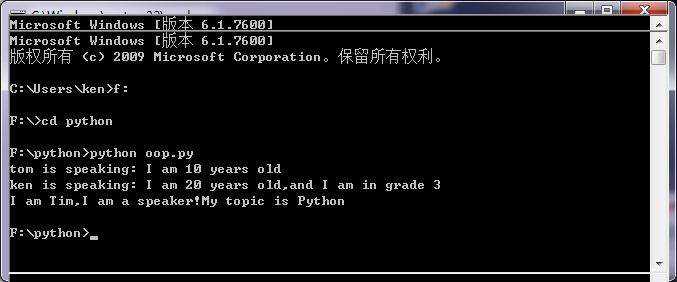python类定义和类继承详解

class :

__private_attrs 两个下划线开头，声明该属性为私有，不能在类地外部被使用或直接访问

__private_method 两个下划线开头，声明该方法为私有方法，不能在类地外部调用

__init__ 构造函数，在生成对象时调用
__del__ 析构函数，释放对象时使用
__repr__ 打印，转换
__setitem__按照索引赋值
__getitem__按照索引获取值
__len__获得长度
__cmp__比较运算
__call__函数调用

__sub__减运算
__mul__乘运算
__p__除运算
__mod__求余运算
__pow__称方

#类定义
class people:
#定义基本属性
name = ”
age = 0
#定义私有属性,私有属性在类外部无法直接进行访问
__weight = 0
#定义构造方法
def __init__(self,n,a,w):
self.name = n
self.age = a
self.__weight = w
def speak(self):
print(“%s is speaking: i am %d years old” %(self.name,self.age))
p = people(‘tom’,10,30)
p.speak()

1.单继承

class (父类名)

eg.
class childbook(book)
age = 10

2.类的多重继承

class 类名(父类1,父类2,….,父类n)

#另一个类，多重继承之前的准备
class speaker():
topic = ”
name = ”
def __init__(self,n,t):
self.name = n
self.topic = t
def speak(self):
print(“i am %s,i am a speaker!my topic is %s”%(self.name,self.topic))
#多重继承
class sample(speaker,student):
a =”
def __init__(self,n,a,w,g,t):
student.__init__(self,n,a,w,g)
speaker.__init__(self,n,t)
test = sample(“tim”,25,80,4,”python”)
test.speak()#方法名同，默认调用的是在括号中排前地父类的方法

def 方法名与父类一致

import a
l = a.类()Posted in 未分类